# Polynomial Construction from Sequences VII

Hello again. Here's where we left off: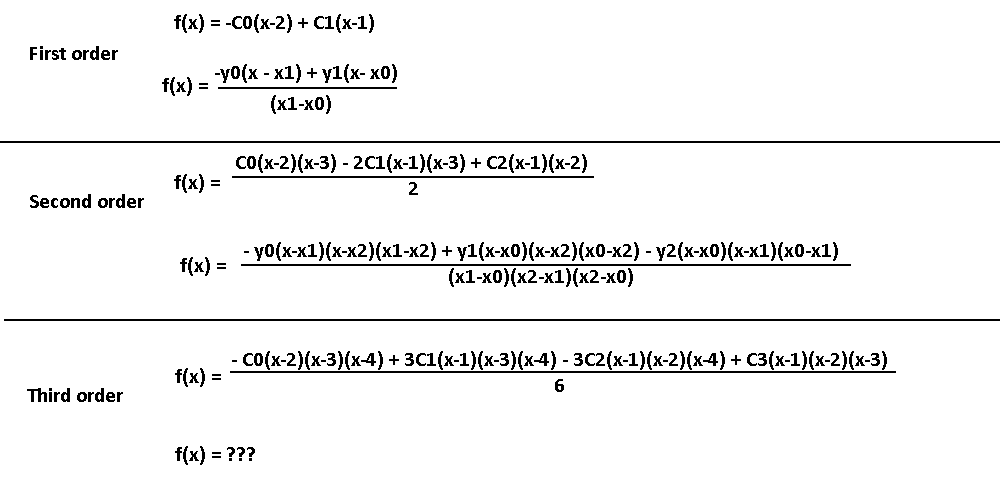We found the second order form for 3 points. Can we figure out the 4 point one without needing to do algebra? Well, we don't have a choice, because I am NOT doing ridiculous amounts of algebra again.

Those extra terms (x1-x2), (x0-x2), and (x0-x1) were unexpected additions to the equation; that's going to make it harder to predict how the third-order form behaves. Here's about how much should be guaranteed, based on the patterns found.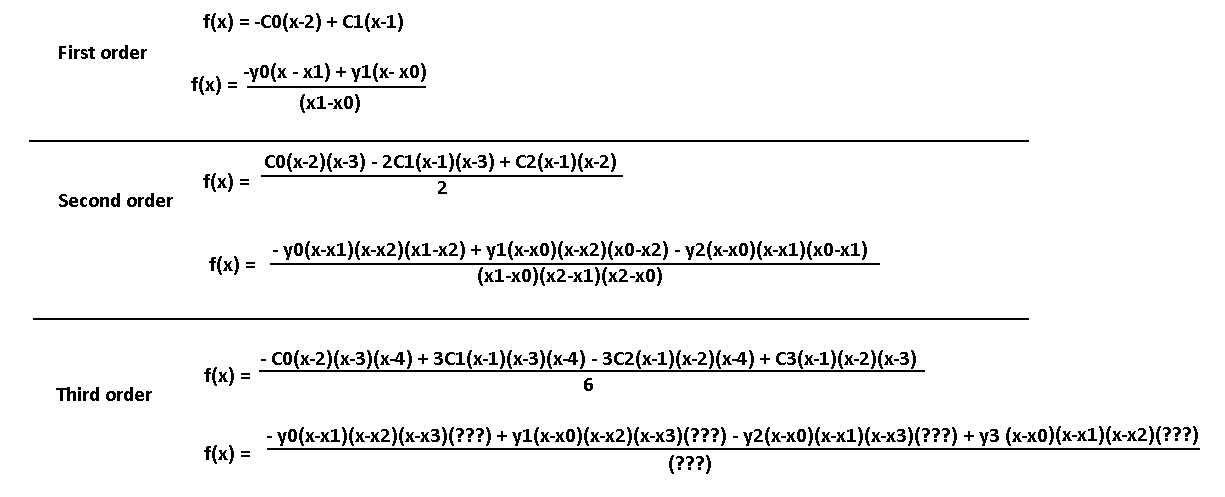Here's a sidenote. It's interesting how nice the factors are, but is there an intuitive way to think about them? You can think of those (x-xN) factors as filters. Take (x-x0) for example. Notice how every y term has it except for y0. This means that when x = x0, all the other y terms will have (x0 - x0) = 0, making that term disappear. This leaves just the y0 term left in the numerator. When plugging in x1, all terms disappear except for y1, and so on. This ensures that we get specifically the y value we want for the specific x value we put in, in theory!

We're going to have to be smart on how we figure out these hidden terms, both for the yN values and for the denominator. We can isolate a single term to make it easier to reverse engineer the factors. How? Set every y value to 0 except for one of them, and figure out what polynomial describes those points to have as a reference. Let's isolate y1. So, y0, y2, y3, will be 0. Now what should the x values be?

Remember that we are trying to find the hidden terms in the numerator and the denominator. These hidden terms are very likely to be groups of a constant x term subtracted by another constant x term, such as (x2 - x1). So, what's going to happen is we plug in all the x and y values into our partial solution, then look at the value it spits out. That value should be 1 once we set x1 to something defined (remember that we are isolating y1). If we end up with a value of, say, 6, then we know that the hidden terms must all multiply together to equal to 1/6, so that y1 is 1.

We're going to use another clever trick. If we make sure that all of the hidden terms are co-prime numbers, then we can take a look at the final value generated, figure out its factors, then figure out what combination of x values must have produced those factors! Note that we are not going to set the x values themselves to co-prime numbers: all the combinations of subtracting two x values must be co-prime, instead.

After careful planning I'll define points (0,0), (2,1), (5,0), (16,0) which should achieve that goal the best. we have 2, 5, 16, 5-2 = 3, 16 - 2 = 14, and 16 - 5 = 11. We also avoid the issue of having any of the differences being 1, and it's an issue because 1 doesn't have an effect on any of the factors, so we don't know if the two x terms that subtract to 1 are important to the equation we are trying to find. Since the second point has a factor of 2, like 16 and 14, then there may be some issues, but considering that point P1 will likely not have an x1 in the numerator anyway it should be fine. Let's try it out!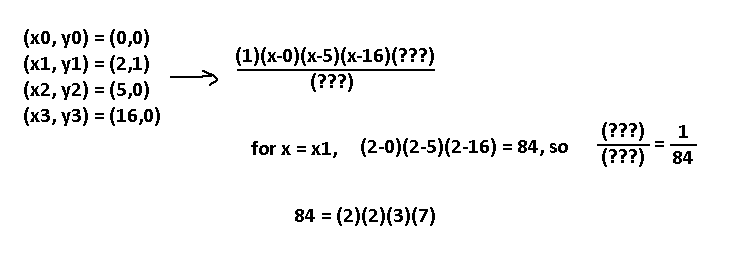So, we plugged in all the points for what parts of the third-order formula we know, and end up with that. Then, set x = x1 and see if the output matches y1, which is 1. We are off by a factor of 1/84, and we can split up 84 into prime factors and figure out what would've generated that value! 2, 2, 3, and… 7? Oh, right, 14 is a multiple of 7. We used 16 - 2 to get 14, which is basically (x3 - x1). That leaves an extra 2 and 3 factor, which can only be generated by 2 - 0 and 5 - 2, respectively: so, (x1 - x0) and (x2 - x1). This means that at the very least, our denominator must include (x1 - x0)(x2 - x1)(x3 - x1). Sweet. We couldn't really find anything with the numerators, because our numerator for 1/84 is 1… that's a bummer.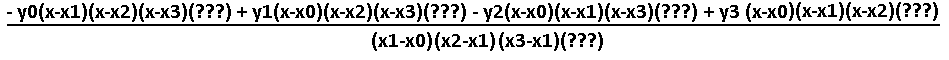Let's try focusing on point 4 this time. Same trick: set all y's to 0 except for y3, then plug in x3 = 16 and see the ratio that comes out. Include the terms we found from before into the denominator.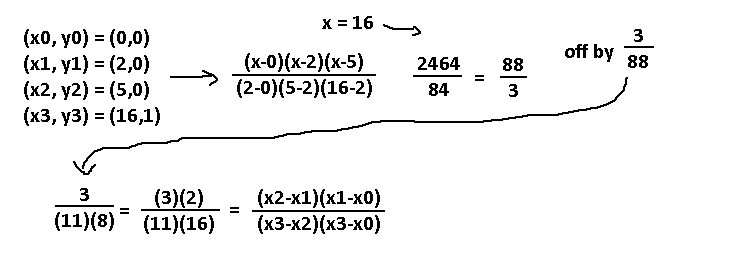A factor of 3/88 is needed. We can split the 88 up into 11 and 8, which is a factor we don't really have as is. We either have to get it by doing 1/2^3 or 2/16. Given how so far none of the extra terms we found had any higher power than 1, I'm going with the theory that it is actually 2/16. From there, four terms were reverse engineered. The two on top go towards the y3 term and the two bottom ones goes for the denominator. Notice how every term shares that same denominator. That means that the terms we found for y1 are going to be messed up, as that factor wouldn't be 1 anymore! We have to cancel out the (x3-x2)(x3-x0) in the denominator with a (x3-x2)(x3-x0) in the numerator for the y1 term, so really we found many terms simultaneously!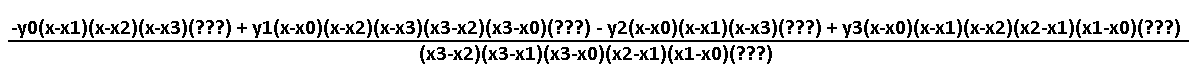Alright, this is starting to look legit. Time to focus on point 1!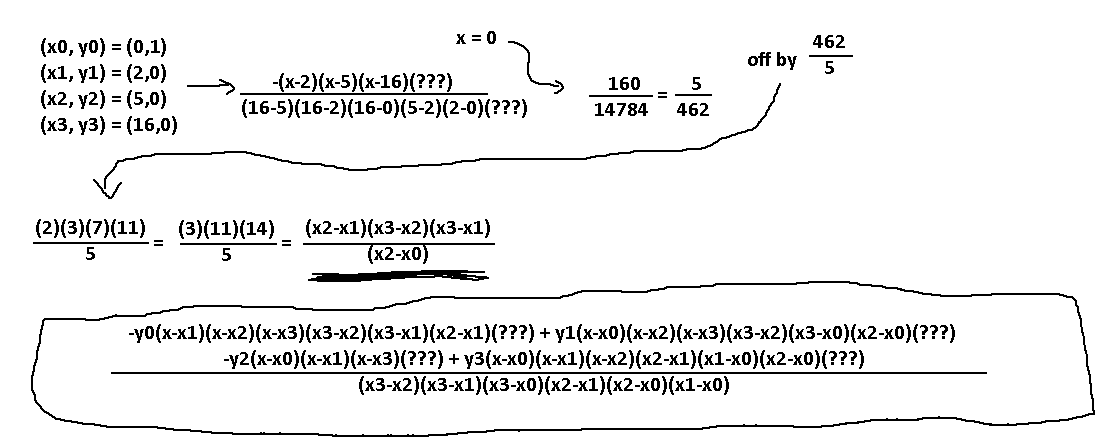I REALLY hope I know what I'm doing here. This is a lot of work. Time for the third point, I guess.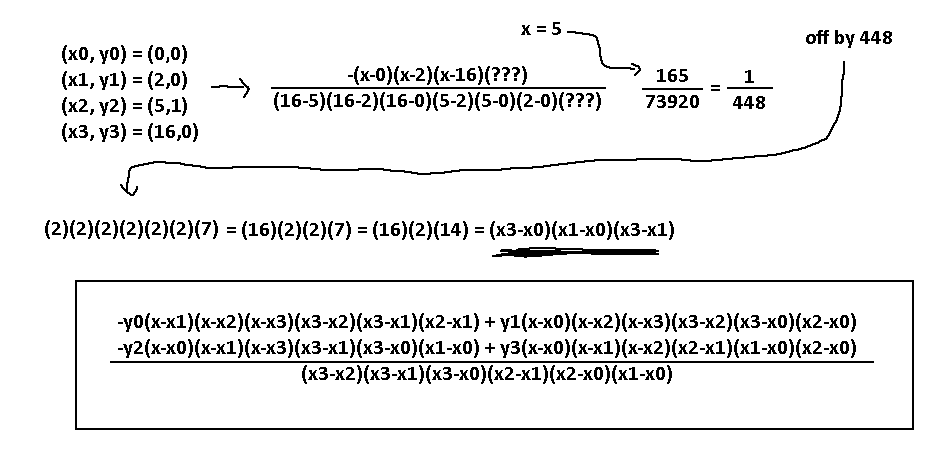This is an excellent sign! After going through all four points we were able to find unique pairs of x's for every y term, and we did not need to include any additional denominator terms this time. If my intuition is correct, this is actually the complete solution to the 4-point problem. Wow, and this is in its most condensed form.

A couple things to note. The denominator has every unique combination of x constant pairs between the four points. They are organized so that the larger xN value is always first. Also, for every yN term, there is never the corresponding xN term in that part. So, y2 never shows an x2 term, for example. Actually, if you think about it like this, every y-term also has every unique combination of x constant pairs, just that their corresponding xN term is not included! You can have 3 unique combinations with 3 points, hence why each y-term has 3 of those factors, and you can have 6 unique combinations with 4 points. We can use this to very easily predict the 5-point problem, now!

Here's the idea: swap signs for every y-term, starting with negative at y0. For every point, calculate the factors for its y by going through all combinations of (x-xN), excluding the x that goes with the y, and also going through all unique pairs of x constants, where the bigger index is first. The denominator is the combination of all unique pairs of x constants for all the points.

Here's what I got for 5 points! Sorry I keep changing the name of these equations, I don't really know what's the best way to call them.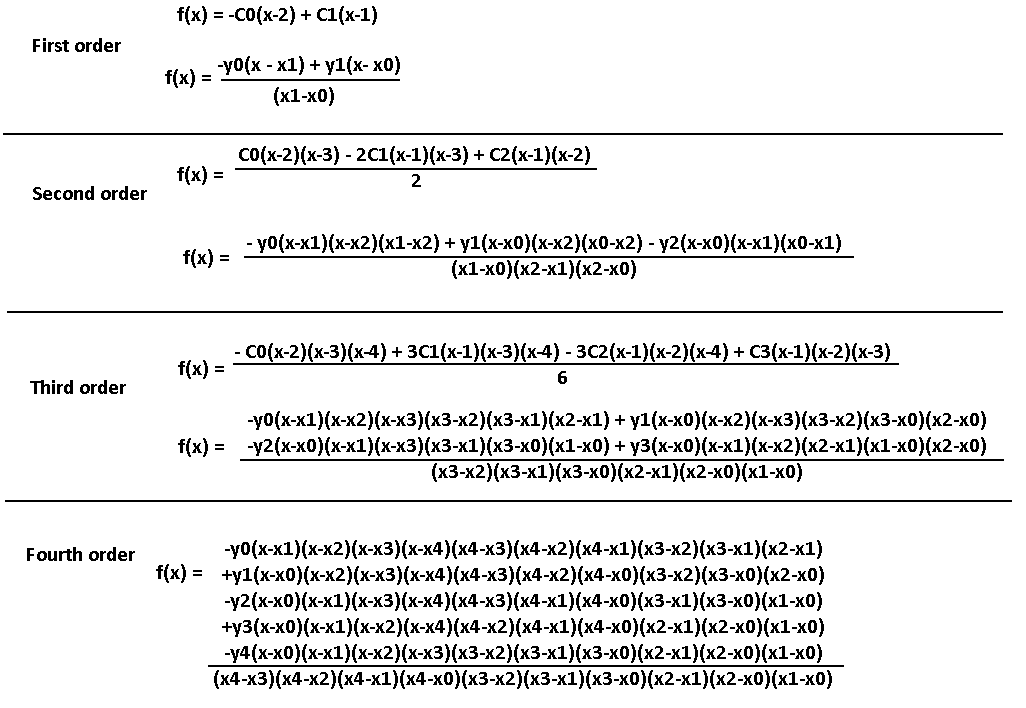Hah! This is so simple, so natural, I don't need to remember much at all to come up with any N-point solution! One thing that sort of bothers me is that the y0 term is always negative, unlike with the other N-term formulas. But, all the terms still alternate in sign, so the only real difference is that the whole expression has a sign change. But which one is right? Figuring out the signs should be the easiest part, so I'll just assume a certain form of alternation and double check that afterwards. Looking way back in my notes, it seems like I ordered the terms so that the lowest xN was first, which may have been the cause of sign changes. Oh well.

It is time. We understand the pattern. Let's get generalizing. Time to bring back the old generalized form for a reference: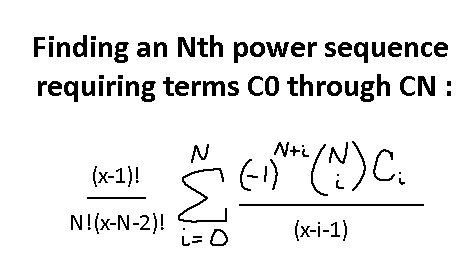Now, our current notations are not going to be good enough for us this time. We are going to have to introduce the Pi notation. It is a relative of Sigma notation, only instead of adding a bunch of terms in a series it will multiply a bunch of terms in the series. Here's an example: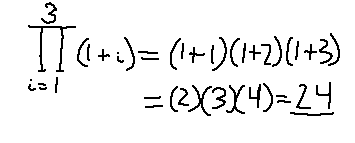This is the only good way we will ever be able to represent the ridiculous number of combinations possible for the pairs of unique x terms. Let's start with the denominator.

We're going to generate them in a specific manner. Since Pi notation will only allow us to count upwards, we will start by generating the "lowest" terms and work our way up. This sequence is what we want:

(x1-x0) (x2-x0) (x2-x1) (x3-x0) (x3-x1) (x3-x2)

Using Pi notation and figuring this out is a lot easier if you already have programming experience, but I'll give out an outline. We need two "loops", one that will count up the right x term and one to count up the left x term. The right x term index always starts at 0 and ends at (i - 1). i is the current index of the left x term, and will continue counting up until we want it to stop generating these terms for a certain N-point problem.

Before we continue, I'm going to set down some definitions. I'm going to stop saying "N-point" and use our first definition of N, which meant the Nth power sequence requiring points P0 through PN. So N = 1 actually means find the linear expression which passes through points P0 and P1, which are (x0, y0), (x1, y1), respectively.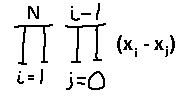That should do for the denominator! Now, we really could just reuse our old generic formula and apply it to the points. The differences are that we know the coefficients are dependent on our x values, so that combination function needs to be replaced. We also should replace the factorial functions with the Pi notation, as we are dealing with variables now. We know what the denominator of the whole thing should be now, so that N! term isn't needed.

Now, how to generate (x-x0)(x-x1)(x-x2), etc… excluding one of the terms? It will be very similar to how we did it before, only we will use Pi notation to control generating terms from 0 to N, and we'll let the sigma notation remove one of the terms for every iteration.

Here's what we have so far: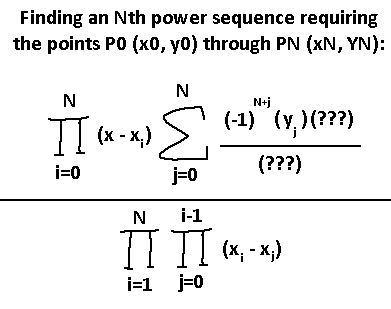Understand so far? The very bottom part is our giant denominator, the top left part is how we generate (x-xN) for every yN term, and the sigma will contain the sign negation, the yN term, the extra x-terms, and the cancelling term for one of the (x-xN)'s in the denominator. Okay.

First, the easy part. Which x-term should be cancelled out? It depends on which yN term we are at, so the j index is involved there. (x - xJ) sounds about right. At yN, exclude any xN terms.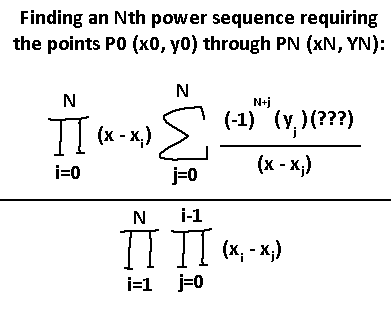Now, the fun part. How are we going to generate the combinations of all the pairs of x-terms after excluding a specific xN? It wouldn't be very efficient, but the simplest way may be to just generate all the combinations like the denominator, then divide out a specific range of those terms to perform the exclusion. For example, for the term y0 for the third order, get our denominator (x3-x2)(x3-x1)(x3-x0)(x2-x1)(x2-x0)(x1-x0). Then, divide out all terms containing x0, so (x3-x0)(x2-x0)(x1-x0). If it was y1, then we'd divide out (x3-x1)(x2-x1)(x1-x0). If it was y2, we'd divide out (x3-x2)(x2-x1)(x2-x0). Hmm.

Screw it. I'm making my own notation for this. Maybe there's some special set notation I can use to help me with this, but instead I'm going to use the denominator formula and apply a constraint to its operation. The following will now mean to exclude a certain iteration from being executed: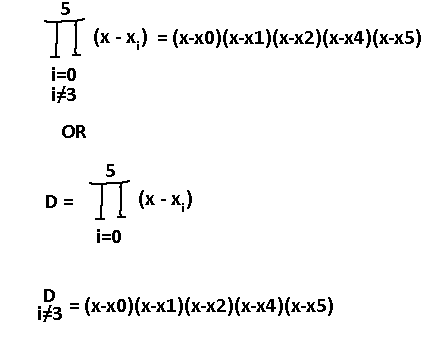Alright, that's going to simplify things. I'm moving the denominator to a variable and I'm going to apply constraints on it when convenient to generate subsets of values. Check this out!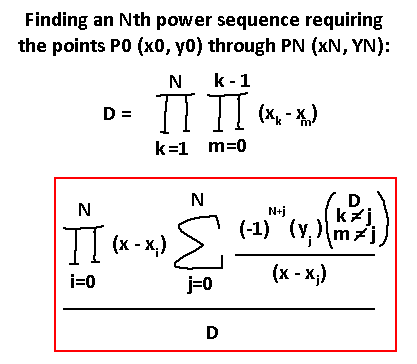Annnnd that's it! I could've moved the top left Pi notation inside the sigma one and done a similar reduction where we exclude a specific x term, but decided not to. Either way, it should mean the same thing. That new D term will exclude any iteration where index J exists when generating D, which relates to yJ. Let's try this out for N = 3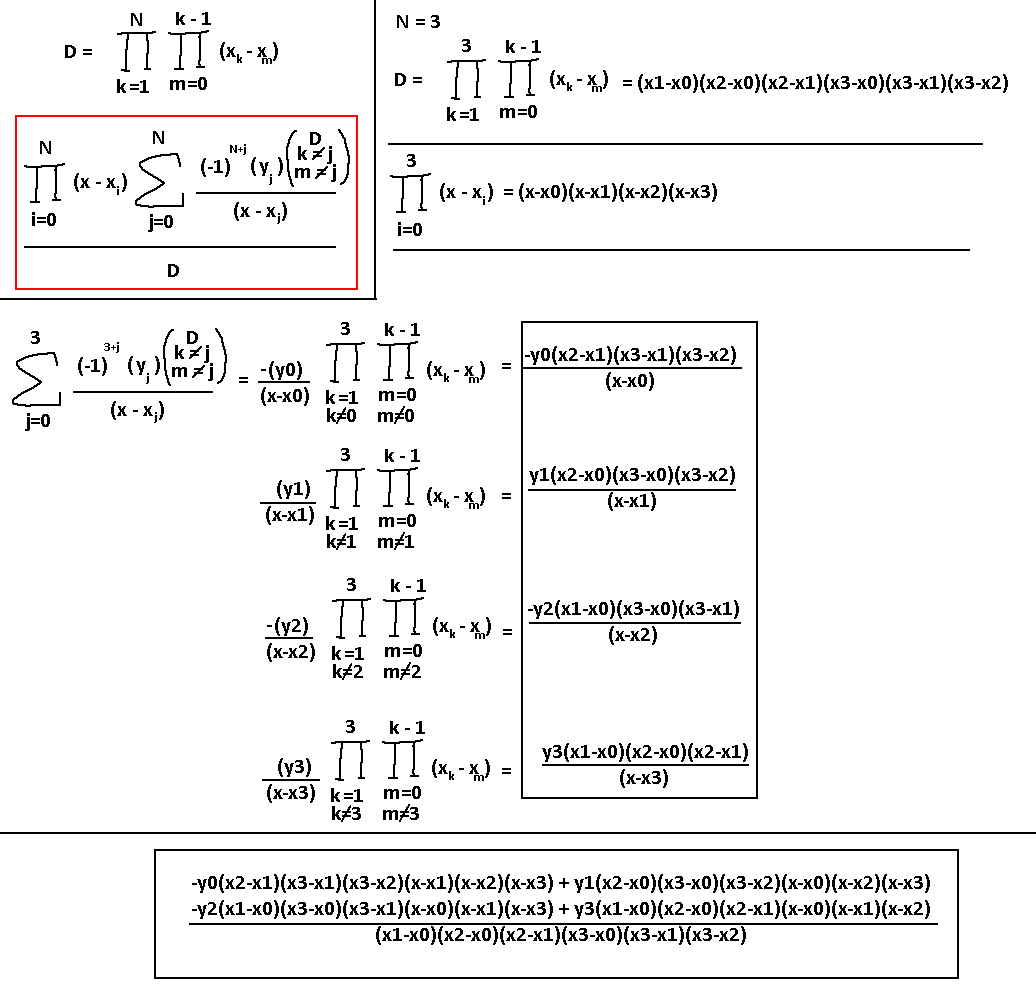I tested out the result and it works for (1,1),(2,4),(6,36), and I get the expected x^2.

So... is there a simpler way to understand this? I have an updated explanation of this below, edited at 11/3/2019 after some playing around with examples.

Let's figure out how to find a polynomial that passes through these points: (2,4), (3,3), (5,7). The trick is to look at one point at a time. Let's start at (2,4). This term will correspond to the x = 2 point, and we must make that term equal 0 when x = 3 and x = 5, the other two x terms defined from our three points. That way, when plugging in those other x's, they won't interfere with the expected y = 4 value we want to get. The best way to do that is to include the factors (x-3)(x-5). For x = 3 or x = 5 the term will be zero.

So (x-3)(x-5) is what we have so far. Plugging in x = 2 for that, we get (-1)(-3) = 3, but the value should be 4. Simply include a 4/3 factor and we're done! The first term is now (4/3)(x-3)(x-5).

The next point is (3,3). If we want the next term to zero out when plugging in x = 2 and x = 5, then the factors should be (x-2)(x-5). Notice that this term will not interfere with the first term we made, because of the (x-2) factor. Plug in x = 3 to this, and we get (1)(-2) = -2. The value we want is y = 3, so add in a (3/-2) factor. This means the second term is (-3/2)(x-2)(x-5).

Finally, theres (5,7). Remove interference with the other two points with (x-2)(x-3). Plug in x = 5 and we get (3)(2) = 6. The value we want is y = 7, so use a (7/6) factor, for a third term of (7/6)(x-2)(x-3). Therefore, the final formula is (4/3)(x-3)(x-5) + (-3/2)(x-2)(x-5) + (7/6)(x-2)(x-3).

Now that this problem has been fully solved, I have a confession to make… this has already been a solved problem. It has been solved hundreds of years ago! I didn't know, of course, many years back. But, the true challenge was attempting to derive this formula from nothing. If you want to compare this solution to the known solution, look up the Lagrange Interpolating Polynomial. That solution even manages to remove the need for alternating signs.

I'm very impressed if you made it through this whole series with my struggles. This was a problem whose solution I didn't know for over 6 years, and refused to look up the answer until I finally derived the solution myself. Thanks for reading.